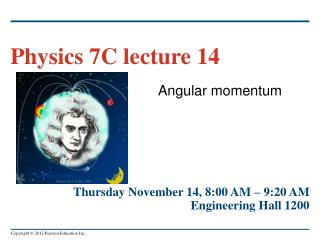DownloadDownload PresentationAngular momentum

# Angular momentum

Télécharger la présentation## Angular momentum

- - - - - - - - - - - - - - - - - - - - - - - - - - - E N D - - - - - - - - - - - - - - - - - - - - - - - - - - -
##### Presentation Transcript

1. Physics 7C lecture 14 • Angular momentum Thursday November 14, 8:00 AM – 9:20 AM Engineering Hall 1200

2. Torque as a vector • Torque can be expressed as a vector using the vector product. • How to find the direction of torque using a right hand rule.

3. Rigid body rotation about a moving axis • The motion of a rigid body is a combination of translational motion of the center of mass and rotation about the center of mass • The kinetic energy of a rotating and translating rigid body is K = 1/2 Mvcm2 + 1/2 Icm2.

4. Rolling without slipping • The condition for rolling without slipping is vcm = R. • Figure 10.13 shows the combined motion of points on a rolling wheel.

5. Work and power in rotational motion • The total work done on a body by the torque is equal to the change in rotational kinetic energy of the body and the power due to a torque is P = zz.

6. Angular momentum • What’s the analogue of momentum P for angular motion?

7. Angular momentum • Momentum : p = m v • angular momentum: τ = r × p = m r × v

8. Angular momentum • The angular momentum of a rigid body rotating about a symmetry axis is parallel to the angular velocity and is given by L = I. • Right hand rule!  

9. Angular momentum • For any system of particles  = dL/dt. • For a rigid body rotating about the z-axis z = Iz. • It makes sense!

10. Conservation of angular momentum • When the net external torque acting on a system is zero, the total angular momentum of the system is constant (conserved).

11. Conservation of angular momentum

12. Q10.11 A spinning figure skater pulls his arms in as he rotates on the ice. As he pulls his arms in, what happens to his angular momentum L and kinetic energy K? A. L and K both increase. B. L stays the same; K increases. C. L increases; K stays the same. D. L and K both stay the same.

13. A10.11 A spinning figure skater pulls his arms in as he rotates on the ice. As he pulls his arms in, what happens to his angular momentum L and kinetic energy K? A. L and K both increase. B. L stays the same; K increases. C. L increases; K stays the same. D. L and K both stay the same.

14. Conservation of angular momentum

15. A rotational “collision” • Find ω. Where is the energy lost?

16. Angular momentum in a crime bust • A bullet hits a door causing it to swing. Find ω.

17. Angular momentum in a crime bust

18. Gyroscopes and precession • For a gyroscope, the axis of rotation changes direction. The motion of this axis is called precession.

19. Non-rotating gyro

20. A rotating flywheel • For a spinning flywheel, the magnitude of the angular momentum stays the same, but its direction changes continuously.

21. A rotating flywheel • precession angular frequency: Ω = τ / L.

22. A precessing gyroscopic • What is the precession direction? CW or CCW?